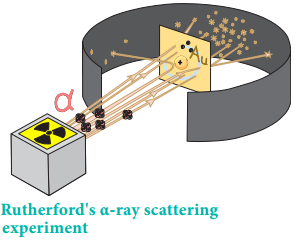Find free online Chemistry Topics covering a broad range of concepts from research institutes around the world.

## Introduction to Atom Models

Let us recall the history of the development of atomic models from the previous classes. We know that all things are made of matter. The basic unit that makes up all matter is atom. The word ‘atom’ has been derived from the Greek word ‘a – tomio’ meaning nondivisible.

Atom was considered as nondivisible until the discovery of subatomic particles such as electron, proton and neutron. J. J. Thomson’s cathode ray experiment revealed that atoms consist of negatively charged particles called electrons.

He proposed that atom is a positively charged sphere in which these electrons are embedded like the seeds in the watermelon. Later, Rutherford’s α-ray scattering experiment results proved that Thomson’s model was wrong. Rutherford bombarded a thin gold foil with a stream of fast moving α-particles. It was observed that

1. Most of the α-particles passed through the foil
2. Some of them were deflected through a small angle and
3. Very few α-particles were reflected back by 180°Based on these observations, he proposed that in an atom there is a tiny positively charged nucleus and the electrons are moving around the nucleus with high speed. The theory of electromagnetic radiation states that a moving charged particle should continuously loose its energy in the form of radiation.

Therefore, the moving electron in an atom should continuously loose its energy and finally collide with nucleus resulting in the collapse of the atom. However, this doesn’t happen and the atoms are stable. Moreover, this model does not explain the distribution of electrons around the nucleus and their energies.

Bohr Atom Model:

The work of Planck and Einstein showed that the energy of electromagnetic radiation is quantised in units of hν (where ν is the frequency of radiation and h is Planck’s constant 6.626 × 10-34 Js). Extending Planck’s quantum hypothesis to the energies of atoms, Niels Bohr proposed a new atomic model for the hydrogen atom. This model is based on the following assumptions:

1. The energies of electrons in an atom are quantised.

2. The electron is revolving around the nucleus in a certain circular path of fixed energy called stationary orbit.

3. Electron can revolve only in those orbits in which the angular momentum (mvr) of the electron must be equal to an integral multiple of h/2π.

i.e. mvr = nh/2π ……………… (2.1)
where n = 1, 2, 3, … etc.,

4. As long as an electron revolves in the fixed stationary orbit, it doesn’t lose its energy. However, when an electron jumps from higher energy state (E2) to a lower energy state (E1), the excess energy is emitted as radiation. The frequency of the emitted radiation is

E2 – E1 = hυ
and
υ = $$\frac{(E2)-E1)}{h}$$ ………………. (2.2)

Conversely, when suitable energy is supplied to an electron, it will jump from lower energy orbit to a higher energy orbit.

Applying Bohr’s postulates to a hydrogen like atom (one electron species such as H, He+ and Li2+ etc..) the radius of the nth orbit and the energy of the electron revolving in the nth orbit were derived.

The results are as follows:The detailed derivation of rn and En will be discussed in 12th standard atomic physics unit.

Limitation of Bohr’s Atom Model:

The Bohr’s atom model is applicable only to species having one electron such as hydrogen, Li2+ etc… and not applicable to multi electron atoms. It was unable to explain the splitting of spectral lines in the presence of magnetic field (Zeeman effect) or an electric field (Stark effect).

Bohr’s theory was unable to explain why the electron is restricted to revolve around the nucleus in a fixed orbit in which the angular momentum of the electron is equal to nh/2π and a logical answer for this, was provided by Louis de Broglie.